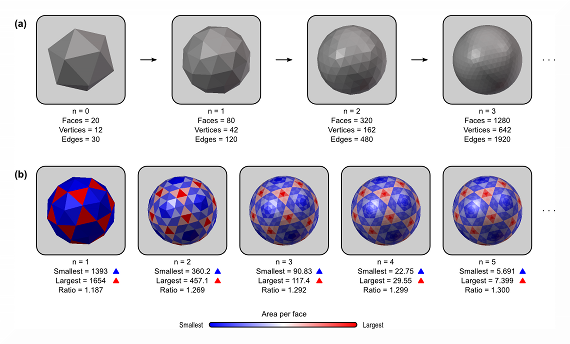# A script to create platonic polyhedra, with recursive subdivision

Suppose you need a tri-dimensional file that contains a platonic polyhedron, say, an octahedron or an icosahedron, stored as a mesh. This file should, however, meet certain criteria: be in an useful format that can be imported into modelling applications, such as Blender, and be easily manipulable using scripts in languages suited for numerical computations, such as Octave or R and perhaps even be manipulated directly from the shell, using, e.g., gawk.

To meet these demands, below is a tool that creates any of the five platonic polyhedra in Wavefront Object format, with the *.obj extension (in ASCII). The user can specify the desired edge length, the area of the face, the total area, the total volume, the radius of the circumscribed sphere, or the radius of the inscribed sphere.

The tool also allows recursive subdivision of the faces of the polyhedra that are made of triangular faces (tetrahedron, octahedron and icosahedron). The subdivision implemented allows geodesic spheres of Class I (Kenner, 1976) to be produced easily. It is also possible to apply affine transformations to the coordinates of the vertices, so that the resulting mesh can be scaled, translated, rotated and sheared in any direction. Finally, it is possible to randomly perturb the vertex positions, so that the mesh becomes irregular, yet preserving the original topology.

In the case of recursive subdivision, it is important to note that not all edges have the same length, and not all faces have the same area. See the figure below for an illustration:(a) A geodesic sphere can be produced from recursive subdivision of a regular icosahedron. At each iteration, the number of faces is quadruplied. (b) After the first iteration the faces no longer have regular sizes, with the largest face being approximately 1.3 times larger than the smallest as n increases.

The formulas to calculate the number of vertices, edges and faces are:

• Number of vertices:$V=4^n(V_0-2)+2$;
• Number of edges:$E=4^nE_0$;
• Number of faces:$F=4^nF_0$;

where$F_0$,$V_0$ and$E_0$ are, respectively, the number of faces, vertices and edges of the polyhedron with triangular faces used for the initial subdivision:

• Tetrahedron:$V_0=4$,$E_0=6$ and$F_0=4$;
• Octahedron:$V_0=6$,$E_0=12$ and$F_0=8$;
• Icosahedron:$V_0=12$,$E_0=30$ and$F_0=20$.

The figure above and the formulas have been discussed by Winkler et al., 2012.

## Main file

The links to the script are below. Either of these will work; choose according to your needs:

• platonic – This is the Octave script. Simply download it, change the first line to point to your correct Octave location, make it executable, and run it directly from the shell. Call it without arguments to obtain usage information.
• platonic.m – This is the same as above, but can be executed from within Octave or MATLAB. Type ‘help platonic’ to obtain usage information.

## Examples

To create an icosahedron with radius 100, recursively subdivided 7 times, if from the shell, use:

```platonic ico7.obj ico sph 7 100
```

or, if from inside Octave/MATLAB, use:

```platonic('ico7.obj','ico','sph',7,100);
```

The outputs from `platonic` are always in Wavefront (.obj) format. To use this icosahedron, e.g., with areal interpolation, it needs to be converted to FreeSurfer ascii format:

```obj2srf ico7.obj > ico7.srf
```

The obj2srf command is available in the package for areal analysis, here.

## 5 thoughts on “A script to create platonic polyhedra, with recursive subdivision”

1.Knut J Bjuland on said:

Could you please add an example on how to produce and ico7.srf with platonic, which is used with area interpretation?

• Sure, I just updated with an example. Hope it helps.